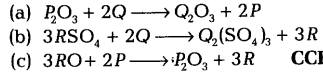# P, Q and R are 3 elements which undergo chemical reactions according to the following equations:

P, Q and R are 3 elements which undergo chemical reactions according to the following equations: